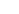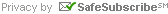Troops

 Green Monkeys Students work on one-to-one correspondence, number recognition, Complements of 10, addition, subtraction, skip counting and developing a life-long love of math Lemurs Students understand basic single digit addition and subtraction through 6 (at a minimum) with little to no use of objects or fingers, comparison of objects, patterns, clock, coins and skip counting. Spider Monkeys Students understand basic single digit addition and subtraction through 18 with little to no use of objects or fingers, adding and subtracting double digits with no carries or borrows, measurement, adding using Complements of 10, missing addends, clock and money. Chimpanzees Students understand Complements of 10 when adding and subtracting, Traditional vs. Mental addition with carries, Borrowing vs. Lending subtraction, time, money, measurement, graphs, line segments, perimeter, small word problems and patterns leading to multiplication Orangutans Students work on different techniques to add, subtract and multiply mentally, an intro to negative numbers, times tables, rounding, estimating, units of measurement, perimeter and area, Magic of 11 and Nikhilam multiplication and in-depth word problem solving. Baboons Students work on different advanced techniques to add, subtract and multiply mentally, subtraction using negative numbers, digit sums to check work, fractions, ratios, decimals, traditional long division, percents, graphing, perimeter and area. Gorillas Students work on different advanced techniques to multiply mentally, solve advanced word problems, decimals, metric, statistics, logic puzzles, and more long division. Apes Students work on algebra, geometry, higher level calculations, exponents, critical thinking skills, advanced word problems and problem solving skills.